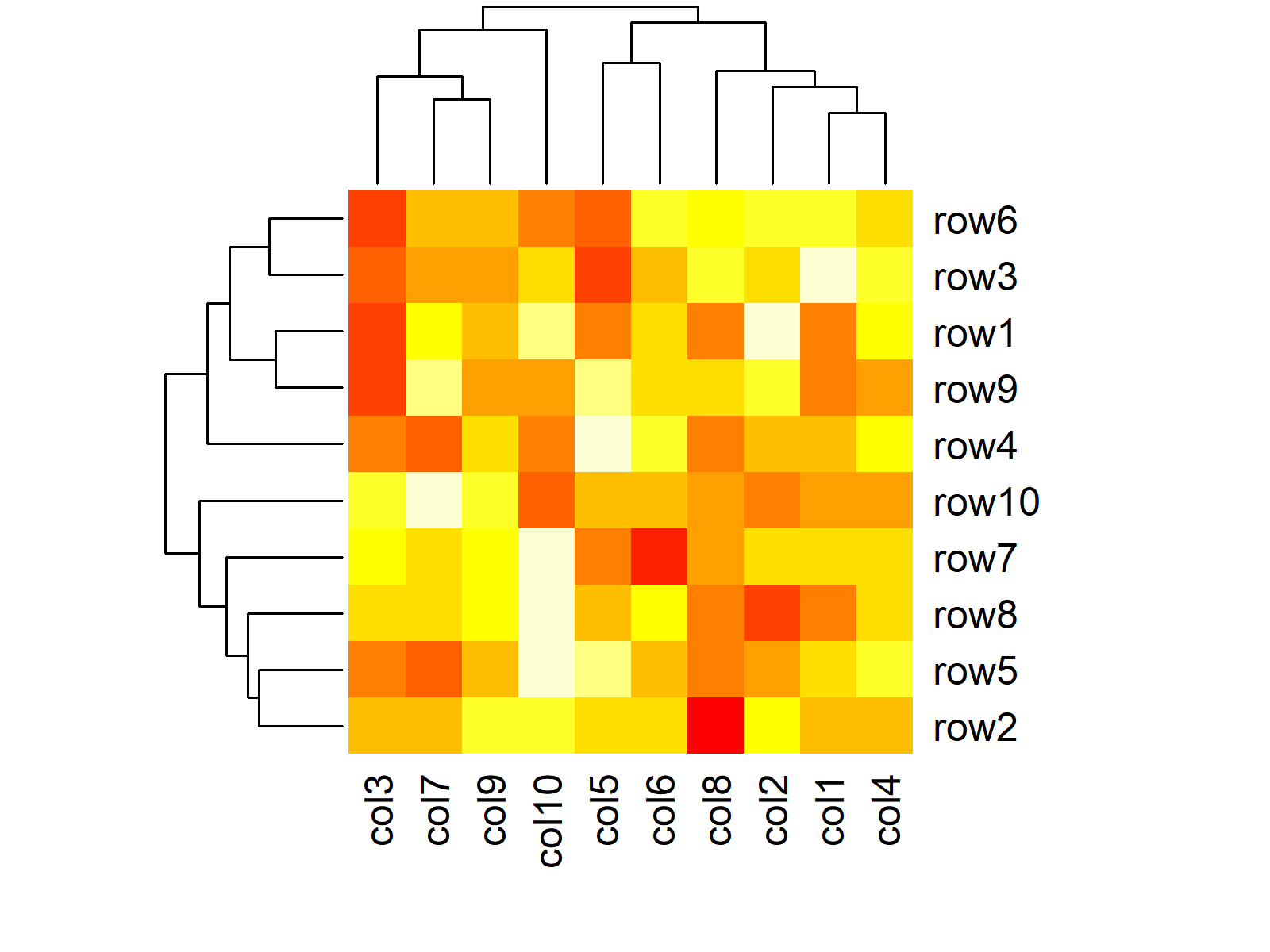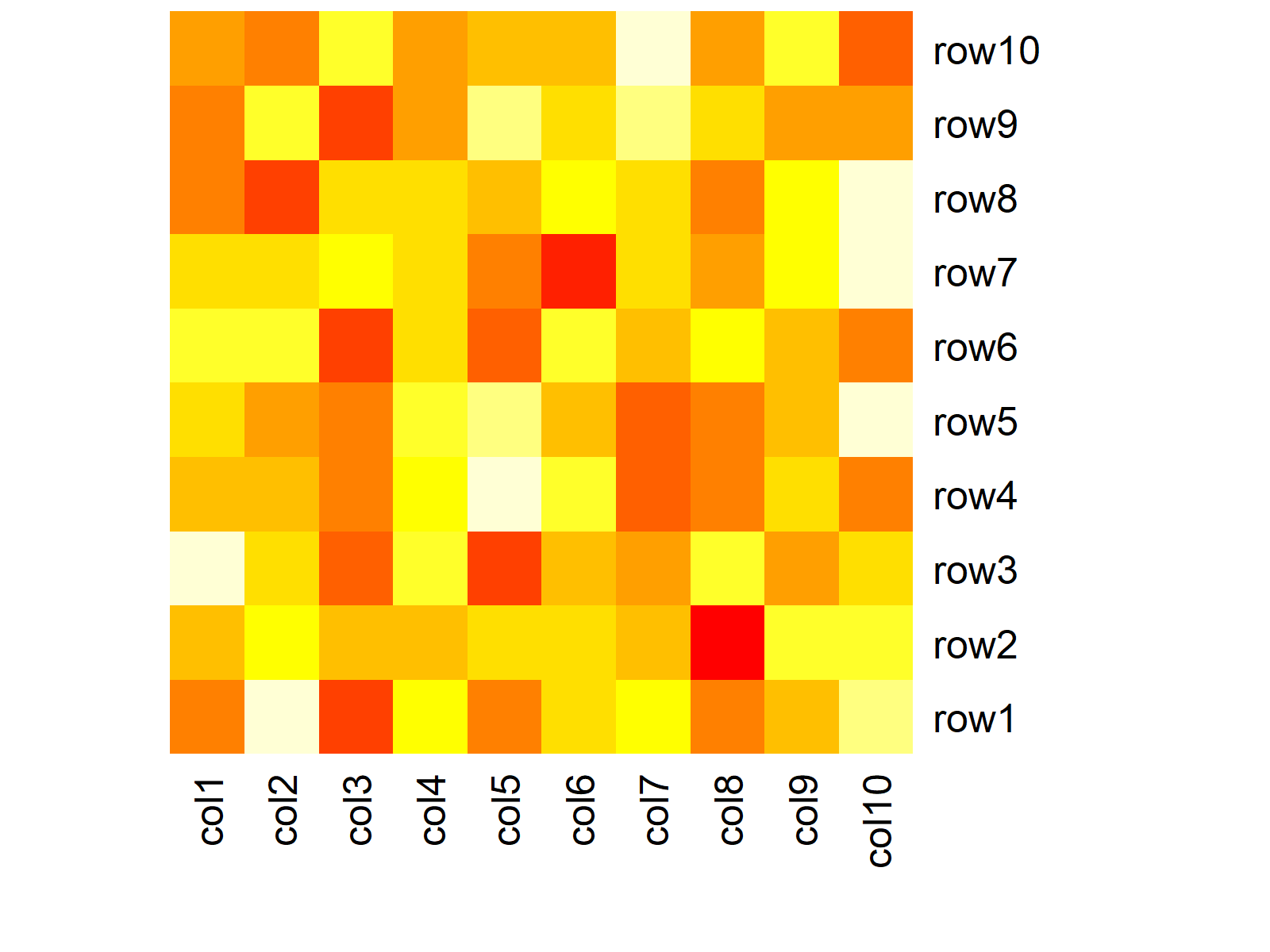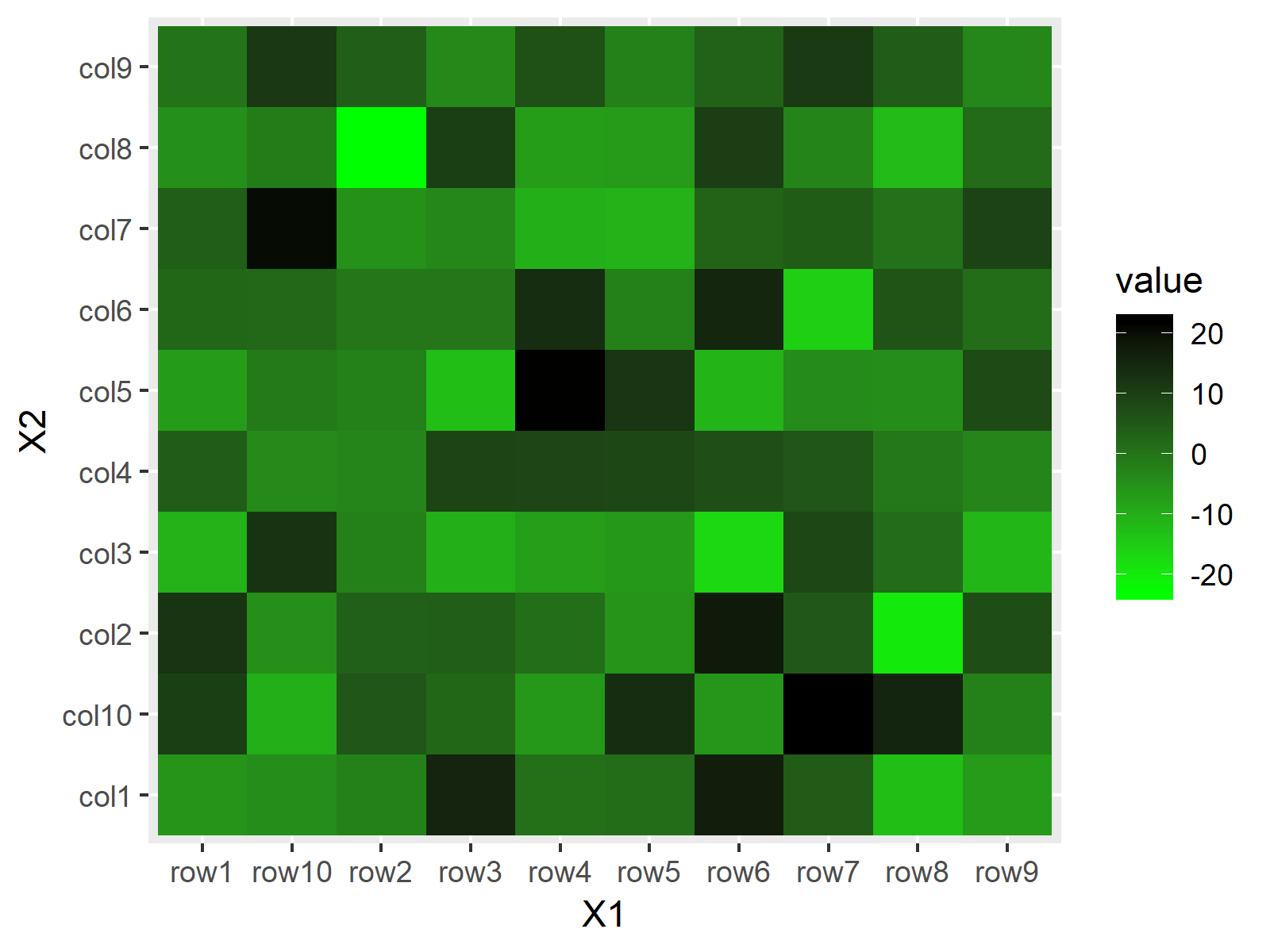# Create Heatmap in R (3 Examples) | Base R, ggplot2 & plotly Package

In this post you’ll learn how to draw heatmaps in the R programming language.

The content of the article is structured as follows:

Let’s dive right in.

## Construction of Example Data

We’ll use the following matrix for the examples of this R tutorial:

```set.seed(123) # Set seed for reproducibility data <- matrix(rnorm(100, 0, 10), nrow = 10, ncol = 10) # Create example data colnames(data) <- paste0("col", 1:10) # Column names rownames(data) <- paste0("row", 1:10) # Row names```

Our data contains ten columns and ten rows with normally distributed random values.

In the following examples, I’ll show how to create heatmaps in R based on different functions and packages. It’s useful to know different ways to create heatmaps, since every package provides a different heatmap design. So keep on reading until the end of the article!

## Example 1: Create Heatmap with heatmap Function [Base R]

The most common function for creating heatmaps in R is the heatmap() function, which is already provided by the base installation of R.

The heatmap function is applied as shown below:

`heatmap(data) # Apply heatmap function`Figure 1: Default Heatmap in Base R.

Figure 1 illustrates the output of the previous R code. By default, the heatmap function returns a heatmap with column and row names as well as a dendogram.

If we want, we can disable the automatically created dendogram:

`heatmap(data, Rowv = NA, Colv = NA) # Remove dendogram`Figure 2: Heatmap without Dendogram in Base R.

Furthermore, we can modify the colors of the heatmap by specifying our own color range with the colorRampPalette function. The following R code produces a function, which creates color ranges between the colors cyan and deeppink3:

`my_colors <- colorRampPalette(c("cyan", "deeppink3")) # Manual color range`

Now, we can create a heatmap with this color range as follows:

`heatmap(data, col = my_colors(100)) # Heatmap with manual colors`Figure 3: Heatmap with Manual Color Range in Base R.

## Example 2: Create Heatmap with geom_tile Function [ggplot2 Package]

As already mentioned in the beginning of this page, many R packages are providing functions for the creation of heatmaps in R.

A popular package for graphics is the ggplot2 package of the tidyverse and in this example I’ll show you how to create a heatmap with ggplot2.

The ggplot2 package requires a long data format. We can create this data format with the reshape package…

```install.packages("reshape") # Install reshape package library("reshape") # Load reshape package```

…and the melt function:

```data_melt <- melt(data) # Reorder data head(data_melt) # First six rows of data # X1 X2 value # row1 col1 -14.15254153 # row2 col1 -6.88199228 # row3 col1 2.44972363 # row4 col1 -0.04076637 # row5 col1 3.54613210 # row6 col1 -2.57462492```

The RStudio console output shows the first 6 rows of our reshaped data. As you can see, the melt function created the two columns X1 and X2, which are containing every possible row and column combination, and a third column with the name value, which is containing the corresponding values.

In order to draw a heatmap with the ggplot2 package, we also need to install and load ggplot2:

```install.packages("ggplot2") # Install ggplot2 package library("ggplot2") # Load ggplot2 package```

Now, we can use the geom_tile function of the ggplot2 package to make a basic heatmap:

```ggp <- ggplot(data_melt, aes(X1, X2)) + # Create heatmap with ggplot2 geom_tile(aes(fill = value)) ggp # Print heatmap```Figure 4: Default Heatmap in ggplot2 Package.

As you can see based on Figure 4, the patter of the heatmap cells is the same as in Base R. However, the general layout is in the typical ggplot2 style.

Of cause, ggplot2 also provides options for the modification of our heatmap. For instance, we can use the scale_fill_gradient function to draw a heatmap with a manual color range:

`ggp + scale_fill_gradient(low = "green", high = "black") # Manual colors of heatmap`Figure 5: Heatmap with Manual Color Range in ggplot2 Package.

## Example 3: Create Heatmap with plot_ly Function [plotly Package]

Another popular package for heatmaps is the plotly package:

```install.packages("plotly") # Install plotly package library("plotly") # Load plotly package```

The plotly package contains the plot_ly function, which can be used to draw a heatmap by specifying type = “heatmap”:

`plot_ly(z = data, type = "heatmap") # Apply plot_ly function`Figure 6: Default Heatmap in plotly Package.

Again, the patter is the same, but the general plot style is different.

The plotly package also provides additional options for the modification of the heatmap. If we want to change the color, we can either specify a color range manually, or we can use some predefined options such as colorscale = “Greys”:

`plot_ly(z = data, colorscale = "Greys", type = "heatmap") # Manual colors`Figure 7: Heatmap with Manual Color Range in plotly Package.

As you can see based on Figure 7, the Greys specification created a heatmap in greyscale.

Note that the plotly package show its graphics in the RStudio viewer instead of the RStudio plot window. For that reason you need to export these plots differently.

Also note that there are many other packages for the creation of heatmaps in R available. In my opinion, however, Base R, ggplot2, and plotly provide the best solutions.

## Video & Further Resources

I have recently published a video on my YouTube channel, which illustrates the R syntax of this article. You can find the video below:

Please accept YouTube cookies to play this video. By accepting you will be accessing content from YouTube, a service provided by an external third party.If you accept this notice, your choice will be saved and the page will refresh.

Also, you might read the other tutorials on my website:

In this R tutorial you learned how to make a heatmap. Don’t hesitate to let me know in the comments below, if you have additional questions.

Subscribe to my free statistics newsletter

•Colin White
October 23, 2019 7:37 pm

Thanks, Joachim, this is a very helpful introduction to heatmaps.

•Joachim
October 24, 2019 5:44 am

Thank you Colin, that’s nice to hear!

•Don Pinkerton
July 28, 2020 1:37 am

This is really helpful, thank you so much! Regarding exporting plotly heatmaps… just what I was looking for by the way, I love the hover-over function… your blog points to a fairly complicated Stack Overflow thread. In R Studio, I just clicked “export” in the viewer… a bunch of stuff was automatically downloaded and I was able to save a fully-functional HTML version of my heatmap. Kinderleicht!

•Joachim
July 28, 2020 5:37 am

Thanks Don Pinkerton, I’m glad that you liked the tutorial! Also, thanks a lot for sharing your way of exporting plotly graphs. I think the thread on Stack Overflow is better if you want to automatize the process. However, for a single export your solution is definitely much simpler to apply. Regards, Joachim

•Patrick Gakuo
August 2, 2020 9:43 pm

This is so well done. Saved me a lot of headache and time

•